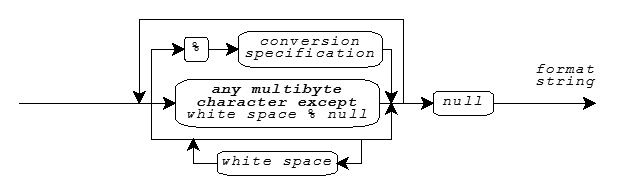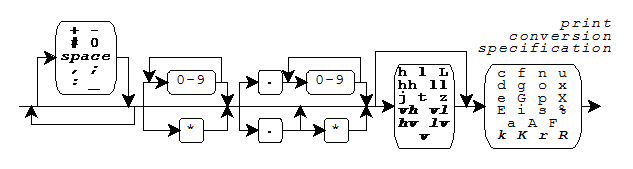# Formatted Output

Print Formats · Print Functions · Print Conversion Specifiers

Several library functions help you convert data values from encoded internal representations to text sequences that are generally readable by people. You provide a format string as the value of the `format` argument to each of these functions, hence the term formatted output. The functions (declared in `<stdio.h>`) convert internal representations to sequences of type char, and help you compose such sequences for display: fprintf, printf, sprintf, vfprintf, vprintf, and vsprintf. For these function, a format string is a multibyte string that begins and ends in the initial shift state.

## Print Formats

A format string has the same syntax for both the print functions and the scan functions, as shown in the diagram.A format string consists of zero or more conversion specifications interspersed with literal text and white space. White space is a sequence of one or more characters `c` for which the call `isspace(c)` returns nonzero. (The characters defined as white space can change when you change the `LC_CTYPE` locale category.) For the print functions, a conversion specification is one of the print conversion specifications described below.

A print function scans the format string once from beginning to end to determine what conversions to perform. Every print function accepts a varying number of arguments, either directly or under control of an argument of type `va_list`. Some print conversion specifications in the format string use the next argument in the list. A print function uses each successive argument no more than once. Trailing arguments can be left unused.

In the description that follows:

• integer conversions are the conversion specifiers that end in `d`, `i`, `o`, `u`, `x`, or `X`
• floating-point conversions are the conversion specifiers that end in `e`, `E`, `f`, `F`, `g`, or `G`

## Print Functions

For the print functions, literal text or white space in a format string generates characters that match the characters in the format string. A print conversion specification typically generates characters by converting the next argument value to a corresponding text sequence. A print conversion specification has the format:Boldface Italic indicates a feature added after C99. Support for fixed-point conversions is added with the C Technical Report TR18037. These closely match the extensions for the Freescale Signal Processing Engine Auxiliary Processing Unit. This implementation also includes, as a conforming extension, conversions for the vectors supported by the Freescale AltiVec architecture.

Following the percent character (`%`) in the format string, you can write zero or more format flags:

• `-` -- to left-justify a conversion
• `+` -- to generate a plus sign for signed values that are positive
• `space` -- to generate a `space` for signed values that have neither a plus nor a minus sign
• `#` -- to prefix `0` on an `o` conversion, to prefix `0x` on an `x` conversion, to prefix `0X` on an `X` conversion, or to generate a decimal point and fraction digits that are otherwise suppressed on a floating-point conversion
• `0` -- to pad a conversion with leading zeros after any sign or prefix, in the absence of a minus (`-`) format flag or a specified precision

For AltiVec vector conversions, you can intersperse format flags with an optional separator, the characters comma, semicolon, equal sign, or underscore. The conversion occurs for each of the elements of the vector, and generates a separator between each pair of elements. If you specify no separator, the default is:

• for the `c` conversion specifier, no character.
• for all other conversion specifications, a space character.

Following any format flags, you can write a field width that specifies the minimum number of characters to generate for the conversion. Unless altered by a format flag, the default behavior is to pad a short conversion on the left with `space` characters. If you write an asterisk (`*`) instead of a decimal number for a field width, then a print function takes the value of the next argument (which must be of type int) as the field width. If the argument value is negative, it supplies a `-` format flag and its magnitude is the field width.

Following any field width, you can write a dot (`.`) followed by a precision that specifies one of the following: the minimum number of digits to generate on an integer conversion; the number of fraction digits to generate on an `e`, `E`, or `f` conversion; the maximum number of significant digits to generate on a `g` or `G` conversion; or the maximum number of characters to generate from a C string on an `s` conversion.

If you write an `*` instead of a decimal number for a precision, a print function takes the value of the next argument (which must be of type int) as the precision. If the argument value is negative, the default precision applies. If you do not write either an `*` or a decimal number following the dot, the precision is zero.

## Print Conversion Specifiers

Following any precision, you must write a one-character print conversion specifier, possibly preceded by a one- or two-character qualifier. Each combination determines the type required of the next argument (if any) and how the library functions alter the argument value before converting it to a text sequence. The integer and floating-point conversions also determine what base to use for the text representation. If a conversion specifier requires a precision p and you do not provide one in the format, then the conversion specifier chooses a default value for the precision.

In the descriptions that follow, array declarations for arguments are not to be taken literally. A declaration of the form:

`    short x`

is shorthand for:

`    struct {short x; }`

And a cast of the form:

`    (short x)x`

is shorthand for:

`    *(struct {short x; } *)&x`

The following table lists all defined combinations and their properties:

```Conversion  Argument                 Converted            Default  Pre-
Specifier    Type                     Value                Base  cision

%c       int x                   (unsigned char)x

%d       int x                   (int)x                   10     1
%hd       int x                   (short)x                 10     1
%ld       long x                  (long)x                  10     1

%e       double x                (double)x                10     6
%Le       long double x           (long double)x           10     6
%E       double x                (double)x                10     6
%LE       long double x           (long double)x           10     6

%f       double x                (double)x                10     6
%Lf       long double x           (long double)x           10     6

%g       double x                (double)x                10     6
%Lg       long double x           (long double)x           10     6
%G       double x                (double)x                10     6
%LG       long double x           (long double)x           10     6

%i       int x                   (int)x                   10     1
%hi       int x                   (short)x                 10     1
%li       long x                  (long)x                  10     1

%n       int *x
%hn       short *x
%ln       long *x

%o       int x                   (unsigned int)x           8     1
%ho       int x                   (unsigned short)x         8     1
%lo       long x                  (unsigned long)x          8     1

%p       void *x                 (void *)x

%s       const char *x           (const char *)x               large

%u       int x                   (unsigned int)x          10     1
%hu       int x                   (unsigned short)x        10     1
%lu       long x                  (unsigned long)x         10     1

%x       int x                   (unsigned int)x          16     1
%hx       int x                   (unsigned short)x        16     1
%lx       long x                  (unsigned long)x         16     1

%X       int x                   (unsigned int)x          16     1
%hX       int x                   (unsigned short)x        16     1
%lX       long x                  (unsigned long)x         16     1

%%       none                    (char)'%'```

The print conversion specifier determines any behavior not summarized in this table. For all floating-point conversions:

• Positive infinity prints as `inf` or `INF`.
• Negative infinity prints as `-inf` or `-INF`.
• Not-a-number (NaN) prints as `nan` or `NAN`.

The upper-case version prints only for an upper-case conversion specifier, such as `%E` but not `%Lg`.

In the following descriptions, p is the precision. Examples follow each of the print conversion specifiers. A single conversion can generate up to 509 characters.

You write `%c` to generate a single character from the converted value.

```    printf("%c", 'a')               generates a
printf("<%3c|%-3c>", 'a', 'b')  generates <  a|b  >```

You write `%d`, `%i`, `%o`, `%u`, `%x`, or `%X` to generate a possibly signed integer representation. `%d` or `%i` specifies signed decimal representation, `%o` unsigned octal, `%u` unsigned decimal, `%x` unsigned hexadecimal using the digits `0-9` and `a-f`, and `%X` unsigned hexadecimal using the digits `0-9` and `A-F`. The conversion generates at least p digits to represent the converted value. If p is zero, a converted value of zero generates no digits.

```    printf("%d %o %x", 31, 31, 31)  generates 31 37 1f
printf("%hu", 0xffff)           generates 65535
printf("%#X %+d", 31, 31)       generates 0X1F +31```

You write `%e` or `%E` to generate a signed decimal fractional representation with a decimal power-of-ten exponent. The generated text takes the form ±d.dddE±dd, where ± is either a plus or minus sign, d is a decimal digit, the decimal point (.) is the decimal point for the current locale, and E is either `e` (for `%e` conversion) or `E` (for `%E` conversion). The generated text has one integer digit, a decimal point if p is nonzero or if you specify the `#` format flag, p fraction digits, and at least two exponent digits. The result is rounded. The value zero has a zero exponent.

```    printf("%e", 31.4)              generates 3.140000e+01
printf("%.2E", 31.4)            generates 3.14E+01```

You write `%f` to generate a signed decimal fractional representation with no exponent. The generated text takes the form ±d.ddd, where ± is either a plus or minus sign, d is a decimal digit, and the decimal point (.) is the decimal point for the current locale. The generated text has at least one integer digit, a decimal point if p is nonzero or if you specify the `#` format flag, and p fraction digits. The result is rounded.

```    printf("%f", 31.4)              generates 31.400000
printf("%.0f %#.0f", 31.0, 31.0)generates 31 31.```

You write `%g` or `%G` to generate a signed decimal fractional representation with or without a decimal power-of-ten exponent, as appropriate. For `%g` conversion, the generated text takes the same form as either `%e` or `%f` conversion. For `%G` conversion, it takes the same form as either `%E` or `%f` conversion. The precision p specifies the number of significant digits generated. (If p is zero, it is changed to 1.) If `%e` conversion would yield an exponent in the range [-4, p), then `%f` conversion occurs instead. The generated text has no trailing zeros in any fraction and has a decimal point only if there are nonzero fraction digits, unless you specify the `#` format flag.

```    printf("%.6g", 31.4)            generates 31.4
printf("%.1g", 31.4)            generates 3.14e+01```

You write `%n` to store the number of characters generated (up to this point in the format) in an integer object whose address is the value of the next successive argument.

`    printf("abc%n", &x)                   stores 3`

You write `%p` to generate an external representation of a pointer to void. The conversion is implementation defined.

`    printf("%p", (void *)&x)        generates, e.g. F4C0`

You write `%s` to generate a sequence of characters from the values stored in the argument C string.

```    printf("%s", "hello")           generates hello
printf("%.2s", "hello")         generates he```

You write `%%` to generate the percent character (`%`).

`    printf("%%")                    generates %`

See also the Table of Contents and the Index.

Copyright © 1992-2006 by P.J. Plauger and Jim Brodie. All rights reserved.